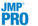Predictive and Specialized Modeling > Naive Bayes > Overview of the Naive Bayes Platform
Publication date: 07/30/2020

#Overview of the Naive Bayes Platform

The Naive Bayes platform classifies observations into classes that are defined by the levels of a categorical response variable. The variables (or factors) that are used for classification are often called features in the data mining literature.

For each class, the naive Bayes algorithm computes the conditional probability of each feature value occurring. If a feature is continuous, its conditional marginal density is estimated. The naive Bayes technique assumes that, within a class, the features are independent. (This is the reason that the technique is referred to as “naive”.) Classification is based on the idea that an observation whose feature values have high conditional probabilities within a certain class has a high probability of belonging to that class. See Hastie et al. (2009).

Because the algorithm estimates only one-dimensional densities or distributions, the algorithm is extremely fast. This makes it suitable for large data sets, and in particular, data sets with large numbers of features. All nonmissing feature values for an observation are used in calculating the conditional probabilities.

Each observation is assigned a naive score for each class. An observation’s naive score for a given class is the proportion of training observations that belong to that class multiplied by the product of the observation’s conditional probabilities. The naive probability that an observation belongs to a class is its naive score for that class divided by the sum of its naive scores across all classes. The observation is assigned to the class for which it has the highest naive probability.

Caution: Because the conditional probabilities of class membership are assumed independent, the naive Bayes estimated probabilities are inefficient.

Naive Bayes requires a large number of training observations to ensure representation for all predictor values and classes. If an observation in the Validation set is being classified and it has a categorical predictor value that was missing in the Training set, then the platform uses the non-missing features to predict. If an observation is missing all predictor values, the predicted response is the most frequent response. The prediction formulas handle missing values by having them contribute nothing to the observation’s score.

For more information about the naive Bayes technique, see Hand et al. (2001), and Shmueli et al. (2010).